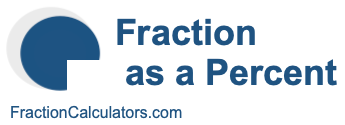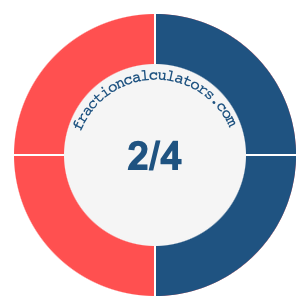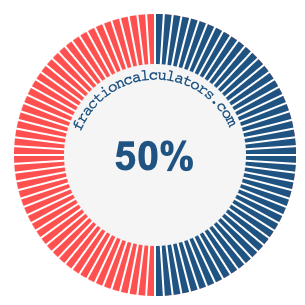2/4 as a percentWhat is 2/4 as a percent? Here we will explain and show you how to calculate and convert 2/4 to a percent.

In addition, we will also illustrate the fraction 2/4 on a pie chart and 2/4 as a percent on a pie chart.

2/4 is a fraction that means 2 out of 4, and percent means something out of 100. We can therefore make the following equation to get 2/4 as a percent:

 2 4
=
 percent 100

To find the percent in the equation above, we multiply 2 by 100 and then divide the product by 4. Here is the solution to the equation above and the answer to 2/4 as a percent:

 2 4
=
 50 100

2/4 = 50%

The illustrations below show you how 2/4 and 50% divide a pie chart differently, but cover the same area because they are the same.Fraction as a Percent Calculator
You can use this tool to convert another fraction to percent.

/

2/5 as a percent
Here is the next fraction on our list that we have converted to a percent for you.